# 2) Quantum Espresso (input file sections)

On this page, I will try to introduce different sections that are in the input file (mostly PW.X file) of the Quantum Espresso (QE) modeling package. This file is like a text file that gives the QE software the necessary parameters and data to start the simulation.

Source: “online course on Computational Materials Physics”, by Prof. Stefaan Cottenier

But before that, let’s look at the numerical methods that can be used for solving the Kohn-Sham equation. If you don’t know what is the Kohn-Sham equation, please go to this page of this website. As a summary:
1. We couldn’t solve the many-body Schrodinger equation and find the energy of the system because of the complexity.
2. Kohn-Sham equation deals with single-particle equation instead of many-body one.
3. By solving the Kohn-Sham equation, we could find the single particle wave function (/phi) and then electron density.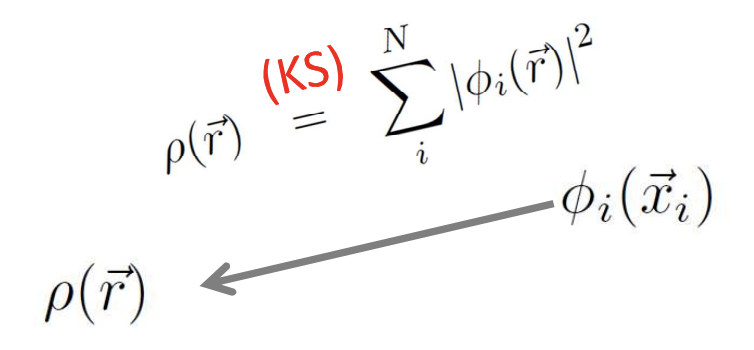4. We said that the ground state energy is a functional of ground-state electron density.
4. Summation of all the energy of all individual particles, plus an unknown exchange-correlation energy, could give us the total energy of the system that we were searching for in the 1st step above.

Now that we know the procedure: How should we solve the Kohn-Sham equation and find the /phi?

To solve the Kohn-Sham equation, we can define: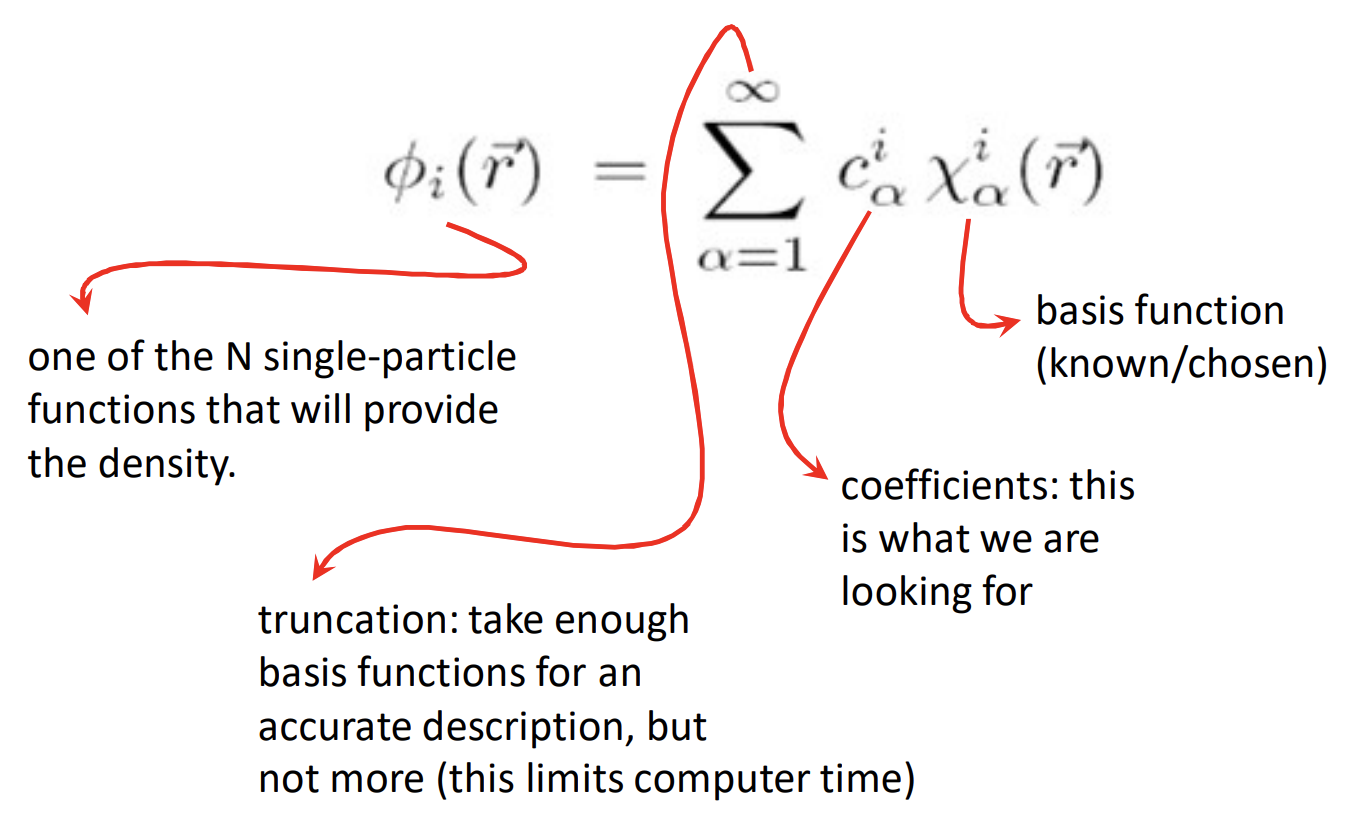Introducing this basis function and their coefficients transform our KS equation into a matrix-algebra equation: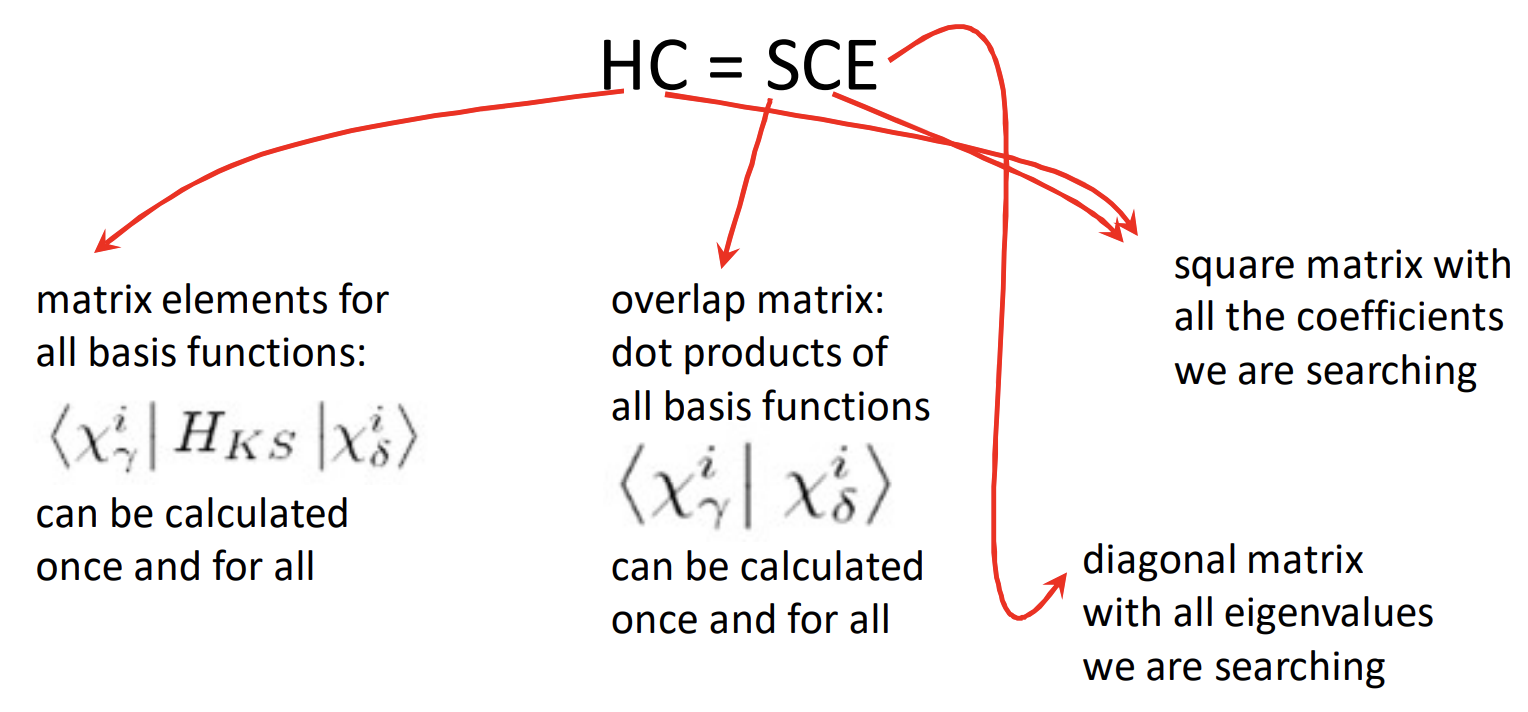So here we will try to find the C matrix that has the coefficients in it. I mean the unknown part is the C, but to solve this equation we also need H_ks that to find it we require the electron density. So here we will remember SCF calculations: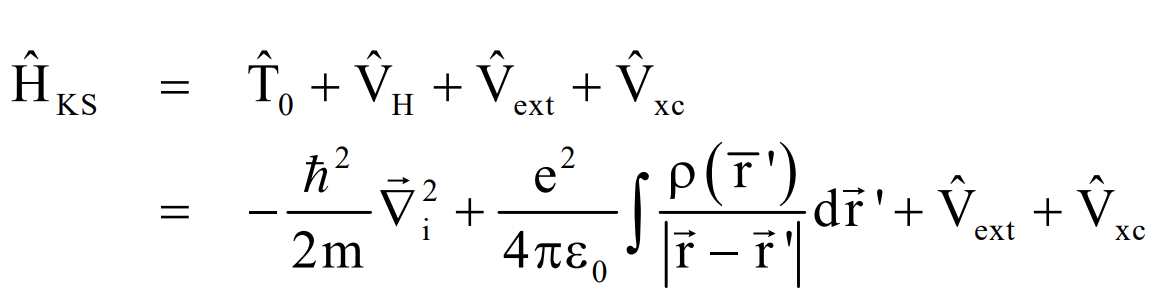SCF: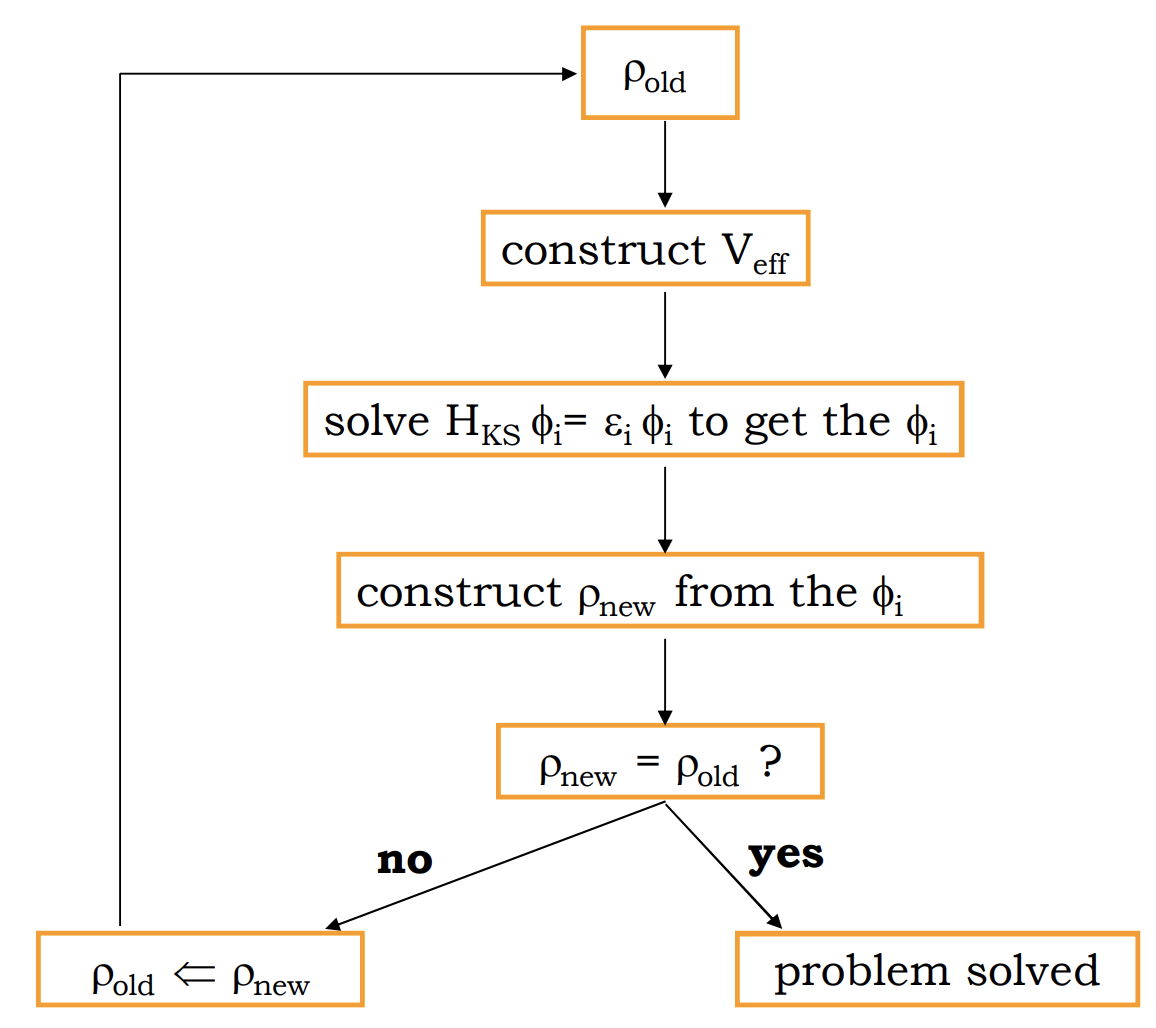Above in the figure in the 3rd step to get the /phi, it uses the matrix equation that we introduced.

Basis functions (/chi,  /ˈk/ ) needs to be chosen. By choosing different kinds of basis functions, we will use different computer codes to find find the /phi and after that electron density. Quantum-Espresso uses plane-wave basis function to simulate the /phi (electron orbital) in companion with pseudopotentials.

In articles, we should determine the basis set size and the k-mesh. for example in this sample article the green parts are the info for basis set size and k-mesh size: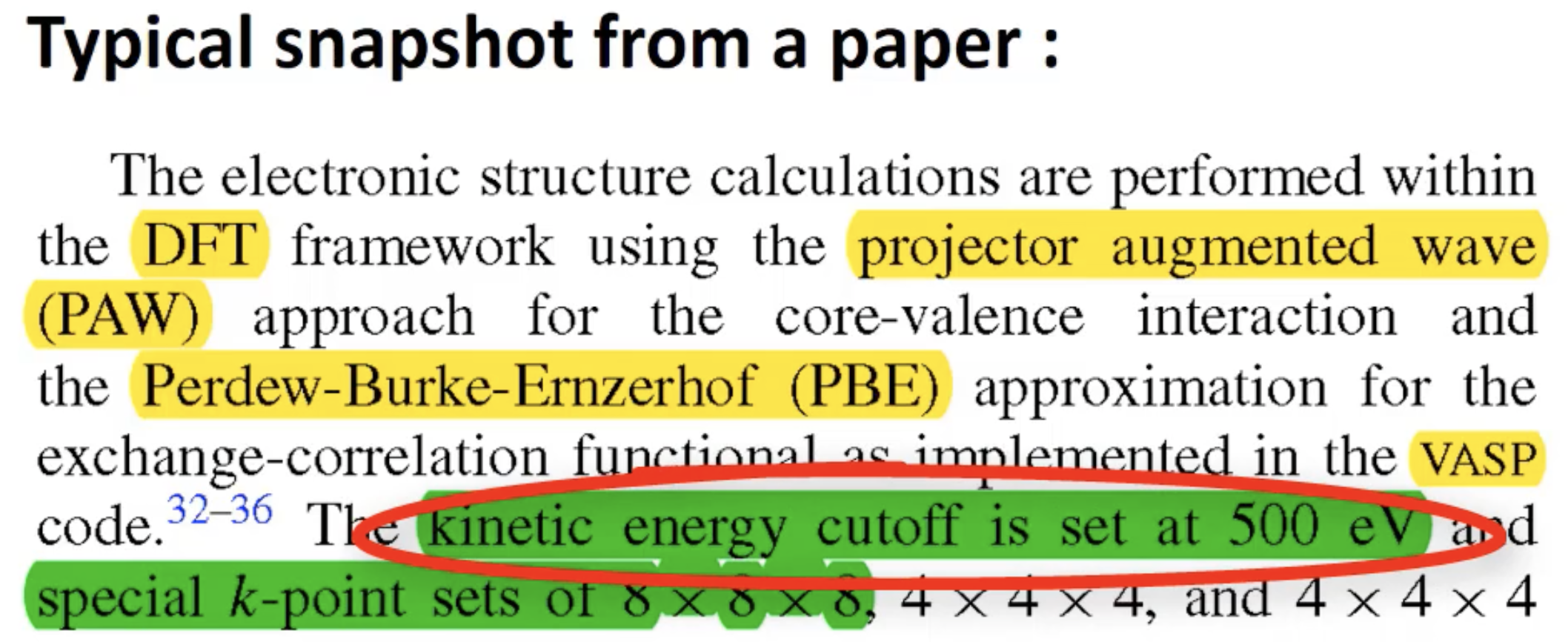`Three important keywords in QE Input files`

QE input file has different sections like this: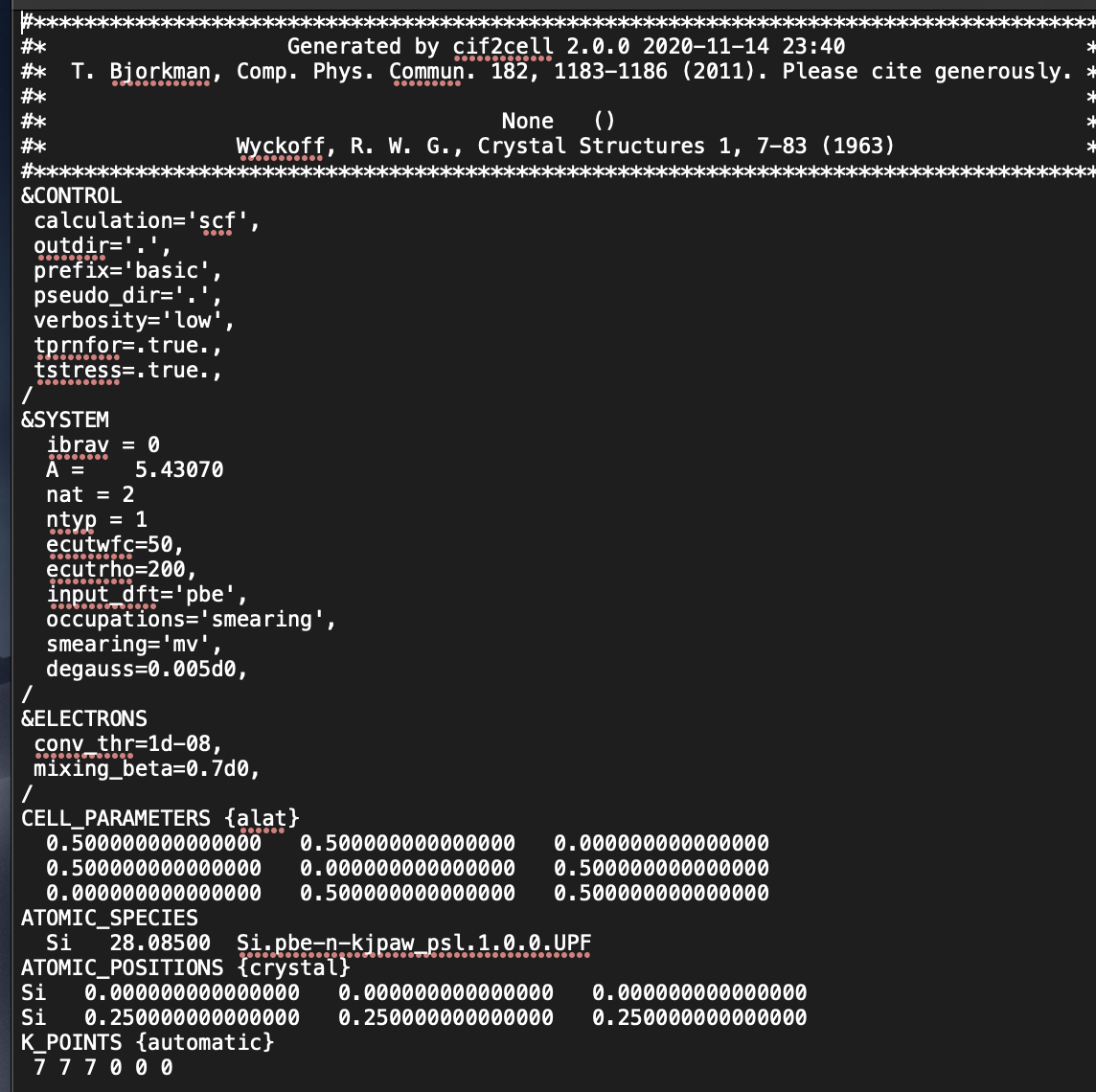1. One of the important keywords that should be defied for your input file is the type of the XC-functional. in the last figure above, under the &SYSTEM section the term:
input_dft=’LDA’, or
input_dft=’pba’,
is the place you choose the XC-functional.

A very important note is that the pseudopotential file should be downloaded from the QE website based on the type of XC-functional that you prefer. When you choose the pseudopotential file, the type of XC-functional is automatically changed in the mentioned line. so you don’t need to write the line above, but you decide which pseudopotential you will use.

For example in the figure above, under the line ATOMIC SPECIES, the pseudopotential file is chosen for Si atoms.

2. The 2nd important keyword is the k-mesh (reciprocal lattice mesh). We obtain many things in DFT by integral over the first Brillouin zone (The lattice cell in reciprocal space). For integration, the code uses numerical method of summation. So we need to define the mesh size and sampling points. k-mesh is expressed in 3 dimensional grid in reciprocal space.

In the input file, under the &ELECTRON section we can find, for example,:
To set a 3x3x3 mesh, use
K_POINTS {automatic}
3  3  3  0  0  0
To use a 5x5x5 mesh, use
K_POINTS {automatic}
5  5  5  0  0  0

3. The 3rd most important keyword in the input file is the basis set size:

As we discussed previously basis sets are used to simulate the Kohn-Sham orbitals (small /phi). It is like expressing a periodic function with infinite consine functions. But we cannot use infinite basis sets to express the KS orbitals. We have to use the maximum number, more than wich we don’t get much more results, and decrease the calculation time

In the input file under the &SYSTEM block, we see, for example:
ecutwfc=30.0d0,
which shows the basis set size to describe the wave function.

Also to describe the density another basis set size is used that is:
ecutrho
by default, it is 4x of ecutwfc but it should be higher if we use PAW pseudopotential, or much larger (8x to12x) for ultrasoft pseudopotential.

After introducing the last 3 keywords to be defined in the input file, let’s decide about running some samples.

Imagine that you have the parameters, atom positions, pseudopotentials and … inside the input file for new crystalline materials. The thing is you need to do a convergence test at first to make sure your future data are meaningful.

How we do the convergence test in QE?
After creating a suitable directory and folders to save your files, download the cif file from crystallographic database and change the format of the file to the one that QE can read.
http://www.crystallography.net/cod/9008678.cif
and the command to change this file to .in file:
cif2cell 9008678.cif -p quantum-espresso -o halite.in
(Note: These commands should be written in Terminal and in the created folder)
The file looks like :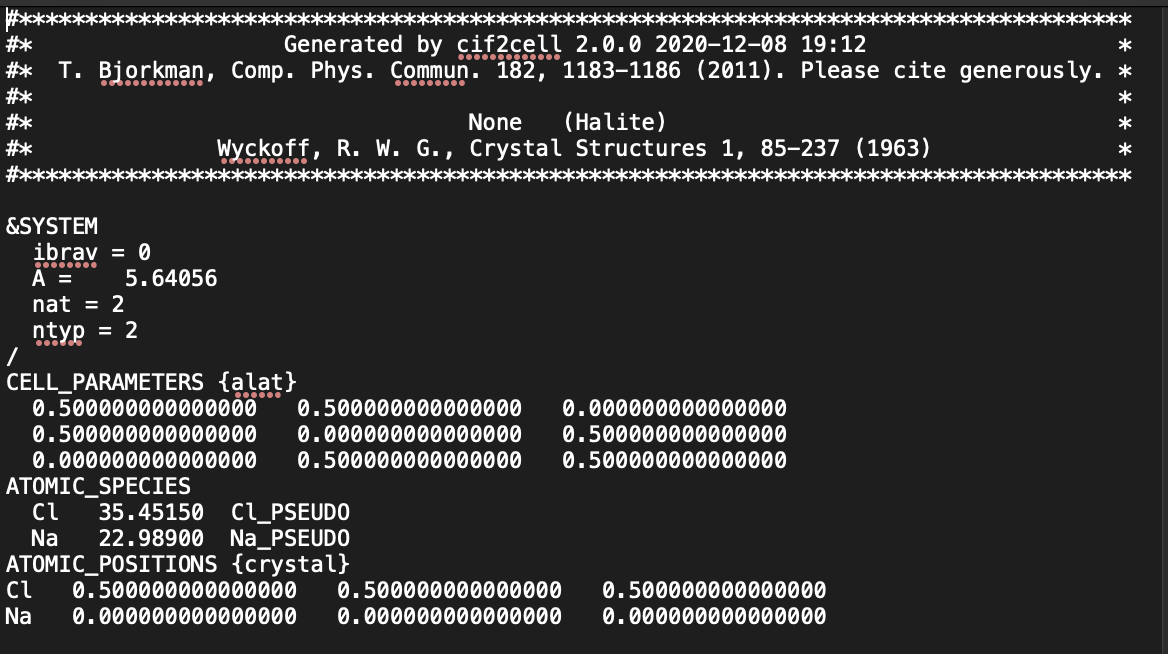Then download the pseudopotential file of Cl and Na from QE website.
Na.pbe-spn-kjpaw_psl.1.0.0.UPF
Cl.pbe-n-kjpaw_psl.1.0.0.UPF

We will add other parts to the input file to become: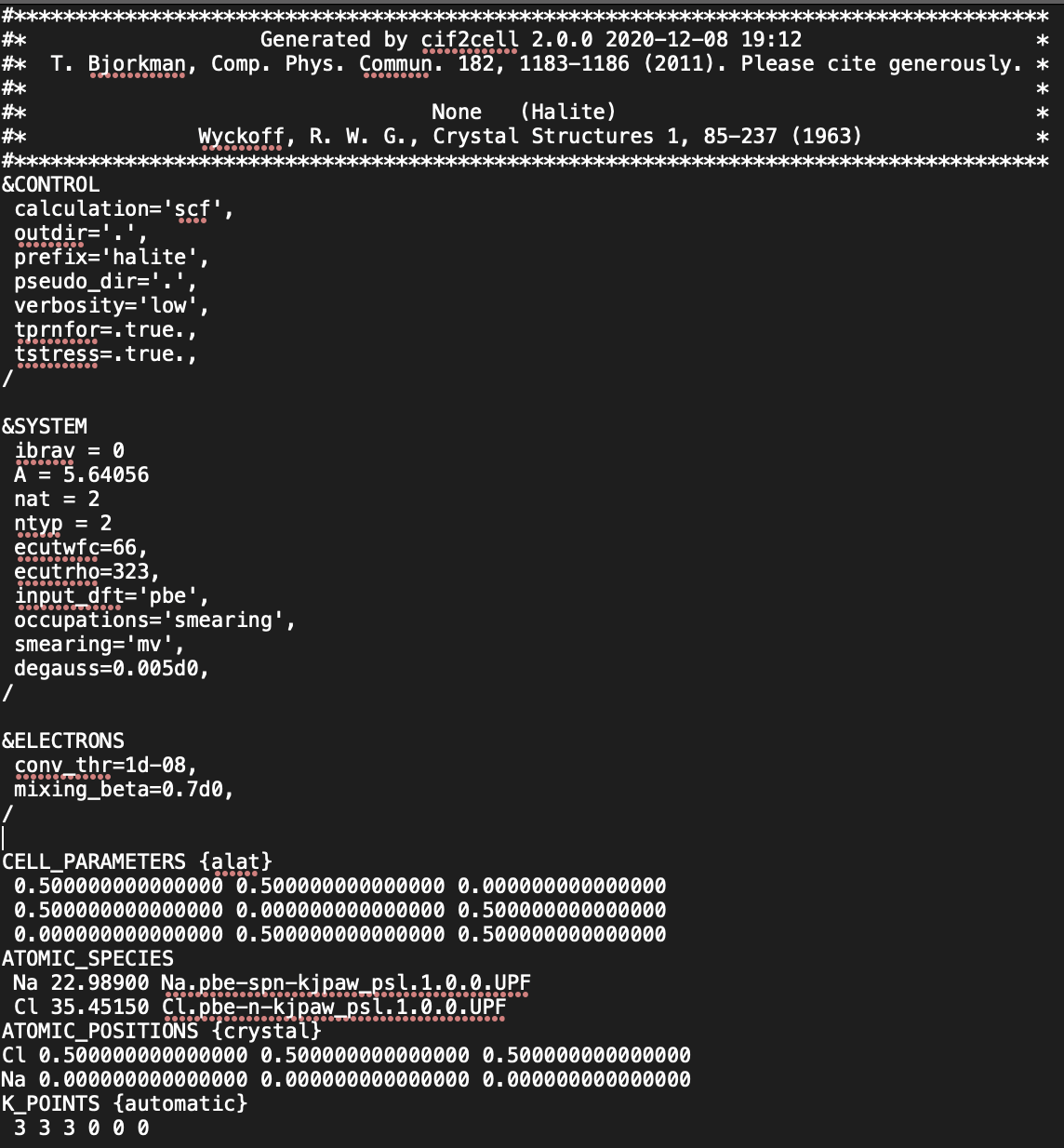As we can see the line:
input_dft=’pbe’,
shows the type exchange correlation potential. We can see also that psuudopotential is also based on this XC potential.

For the basis set size we also look to the pseudopotential file. If we open them we can see the lines for Na and Cl atoms for their own files:
Na-file:
Suggested minimum cutoff for wavefunctions: 66. Ry
Suggested minimum cutoff for charge density: 323. Ry
Cl-file:
Suggested minimum cutoff for wavefunctions: 45.
Ry Suggested minimum cutoff for charge density: 223. Ry
So if we take 66 and 323 for wavefunction and charg density (Maxs of numbers) it would be better.

Practical test

1. For k-mesh choose:
As we said before it is a sampling number in 3D dimension in reciprocal space. It is in &ELECTRONS block. The default is 1x1x1 mesh but we can change it.

When do we decide the k-mesh is enough and there is no bigger sampling size in reciprocal lattice?

Ans: When after some conversion run, the change in the properties does not change that much. We can prepare a table for that.

Which properties do we use to find the optimum mesh size? The most sensitive one to the numbers. It is the hydrostatic pressure.

To run and see the effect of k-mesh on the hydrostatic pressure:

• As mentioned above the input file should be like:
&CONTROL
calculation=’scf’,
outdir=’.’,
prefix=’halite’,
pseudo_dir=’.’,
verbosity=’low’,
tprnfor=.true.,
tstress=.true.,
/&SYSTEM
ibrav = 0
A = 5.64056
nat = 2
ntyp = 2
ecutwfc=66,
ecutrho=323,
input_dft=’pbe’,
occupations=’smearing’,
smearing=’mv’,
degauss=0.005d0,
/&ELECTRONS
conv_thr=1d-08,
mixing_beta=0.7d0,
/CELL_PARAMETERS {alat}
0.500000000000000 0.500000000000000 0.000000000000000
0.500000000000000 0.000000000000000 0.500000000000000
0.000000000000000 0.500000000000000 0.500000000000000
ATOMIC_SPECIES
Na 22.98900 Na.pbe-spn-kjpaw_psl.1.0.0.UPF
Cl 35.45150 Cl.pbe-n-kjpaw_psl.1.0.0.UPF
ATOMIC_POSITIONS {crystal}
Cl 0.500000000000000 0.500000000000000 0.500000000000000
Na 0.000000000000000 0.000000000000000 0.000000000000000
K_POINTS {automatic}
1 1 1 0 0 0
• Open the terminal in the folder containing the input file and write this command line:
pw.x -input basic.in > basic.out
in my system (Mac OS) I have to write this:
mpirun –allow-run-as-root -np 4 pw.x -input halite.in > halite.out
This is bcz I want to use all the CPU cores and also allow the program to run as root.
• In the out put file the:
total stress (Ry/bohr**3) (kbar) P= 243.68
• We will go with K-mesh of 3 3 3 0 0 0:
total stress (Ry/bohr**3) (kbar) P= 3.15
• and K-mesh of 5 5 5 0 0 0:
total stress (Ry/bohr**3) (kbar) P= 1.24
• and K-mesh of 7 7 7 0 0 0:
total stress (Ry/bohr**3) (kbar) P= 1.10
• and K-mesh of 9 9 9 0 0 0:
total stress (Ry/bohr**3) (kbar) P= 1.11

We see that the hydrostatic pressure became constant around K-mesh of  7 7 7 0 0 0. We will go with this from now.

2. For choosing the basis-set size:

• we suggested to have ecutwfc=66 and ecutrho=323, with the multiplication factor of 5.
• first we will optimize the ecutwfc and then with the chosen ecutwfc we will optimize the multiplication factor to optimize ecutrho.
• ecutwfc=16, ecutrho=80, total stress (Ry/bohr**3) (kbar) P= -5324.52
• ecutwfc=26, ecutrho=130, total stress (Ry/bohr**3) (kbar) P= -865.62
• ecutwfc=36, ecutrho=180, total stress (Ry/bohr**3) (kbar) P= -268.15
• ecutwfc=46, ecutrho=230, total stress (Ry/bohr**3) (kbar) P= -8.99
• ecutwfc=56, ecutrho=280, total stress (Ry/bohr**3) (kbar) P= 4.34
• ecutwfc=66, ecutrho=330, total stress (Ry/bohr**3) (kbar) P= 0.95
• ecutwfc=76, ecutrho=380, total stress (Ry/bohr**3) (kbar) P= 4.56
• ecutwfc=86, ecutrho=430, total stress (Ry/bohr**3) (kbar) P= 7.11
• ecutwfc=96, ecutrho=480, total stress (Ry/bohr**3) (kbar) P=  7.85
• ecutwfc=200, ecutrho=1000, total stress (Ry/bohr**3) (kbar) P= 8.3

You see, P valuse changes alot until forexample ecutwfc=66 which is P = 0.95, not that much differnt to P = 8.3. compare to initial values given to ecutwfc.

Now it is time to optimize ecutrho. So far we used the factor of % to find it. But it needs to be optimized. (Note: in the input file, halite.in, we change the section ecutwfc = 90 and by following factor we give the ecutrho = # value. then write the following command in the Terminal window, under the current path :mpirun -np 4 pw.x -input halite.in > halite.out, then open the output fine, halite.out and find P):

• Factor of 2 and ecutrho = 180 and P = 9.13
• Factor of 3 and ecutrho = 270 and P = 7.9
• Factor of 4 and ecutrho = 360 and P = 7.45
• Factor of 5 and ecutrho = 450 and P = 7.59
• Factor of 6 and ecutrho = 540 and P = 7.72
• Factor of 7 and ecutrho = 630 and P = 7.51
• Factor of 8 and ecutrho = 720 and P = …
• Factor of 9 and ecutrho = 810 and P = …
• Factor of 10 and ecutrho = 900 and P = 7.63

We see that after the factor of 4, numbers are in a specific range. To be safe we use the factor of 5.

Important: For every new calculation, we need to do these parts.